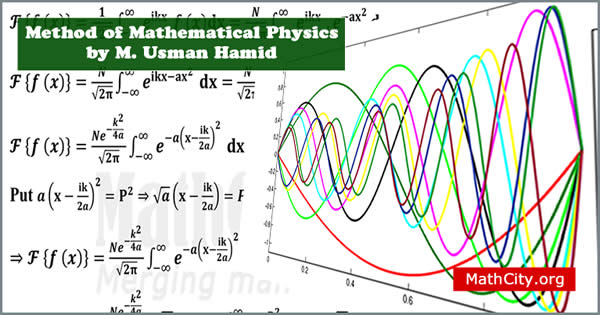# Method of Mathematical Physics by Mr. Muhammad Usman HamidThese notes are send by Mr. Anwar Khan and written by M. Usman Hamid. We are really very thankful to him for providing these notes and appreciates his effort to publish these notes on MathCity.org

 Name Method of Mathematical Physics(MMP) Mr. Muhammad Usman Hamid 250 pages PDF (see Software section for PDF Reader) 4.89 mB

In this section, the main heading are given below.

• Difference equation
• Differential equation
• Ordinary differential equation
• Partial differential equation
• Homogeneous differential equation
• Non-homogeneous differential equation
• The order of a partial differential equation
• The degree of a partial differential equation
• Linear partial differential equation
• Non-linear partial differential equation
• Initial conditions
• Initial value problem
• Boundry conditions
• Boundry value problem
• Principle of superposition
• Eigen value problems
• General forms of first-order linear equations in two variables
• Second-order equations in one independent variable
• Classifiation of second-order linear equations
• Method of seperation of variables
• Strum liouville system (SL-system)
• Sturm liouville (SL) differential operator
• Equation of simple harmonic motion
• Legendre's differential equation
• Bessele's differential equation
• The Hermite equation
• The lagrange equation
• Airy's equation
• Boundary conditions associate with SL system
• Orthogonal function
• Lagrange's identity
• Reality of eigenvalues
• Orthogonality of eigenvalues
• Simplicity of eigenvalues
• Abel's formula
• Completeness of eigenvalues

• msc/notes/method-of-mathematical-physics-m-usman-hamid